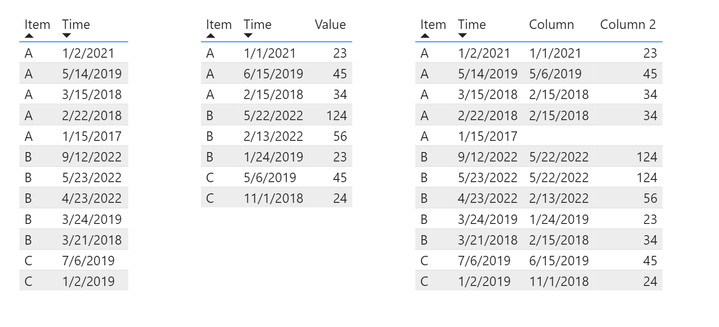cancel
Showing results for
Did you mean:Frequent Visitor

## Get the most recent value from another table with measures

Hello experts,

I ran into this question and hope to get your advice on it.

I have two tables:

Table 1: Item, Date

Table 2: Item, Date, Value

I want to be able to find the most recent date from Table2 that before Table1 date, and also get the value.

I have been tried with Calculated Columns but didn't look right:

Column =
CALCULATE(
MAX(T2[Time]),
FILTER(T2,
T2[Time] <= T1[Time]))

Column 2 =
LOOKUPVALUE(T2[Value],T2[Time],T1[Column])I tried measures but but didn't work well.

Measure =
VAR _maxdate = MAX(T1[Time])
VAR _maxitem = MAX(T1[Item])
RETURN
CALCULATE(
MAX(T2[Value]),FILTER(ALL(T2),T2[Time]<=_maxdate&&T2[Item] =_maxitem)
)

My question is:
How to do this by measures? Otherwise how to do it with calcualted columns?

Many thanks
1 ACCEPTED SOLUTIONSuper User

@XR043 , new column in table 1

MAX(Filter(T2,T2[Time] =t1[Time] &&T2[Item] =T1[Item]), T2[Value])

refer 4 ways (related, relatedtable, lookupvalue, sumx/minx/maxx with filter) to copy data from one table to anotherSuper User

@XR043 , new column in table 1

MAX(Filter(T2,T2[Time] =t1[Time] &&T2[Item] =T1[Item]), T2[Value])

refer 4 ways (related, relatedtable, lookupvalue, sumx/minx/maxx with filter) to copy data from one table to another